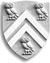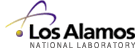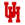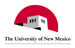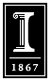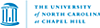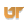# LACSI at Rice University

##### Personal tools
You are here: Home Fixed-Polynomial Approximate Spectral Transformations for Preconditioning the Eigenvalue Problem
##### Document Actions

Heidi K Thornquist (2003)

# Fixed-Polynomial Approximate Spectral Transformations for Preconditioning the Eigenvalue Problem

Master thesis, Rice University, Department of Computational and Applied Mathematics, 6100 Main Street, Houston, TX 77005.

Arnoldi's method is often used to compute a few eigenvalues and eigenvectors of large, sparse matrices. When the eigenvalues of interest are not dominant or well-separated, this method may suffer from slow convergence. Spectral transformations are a common acceleration technique that address this issue by introducing a modified eigenvalue problem that is easier to solve than the original. This modified problem accentuates the eigenvalues of interest, but requires solving a linear system, which is computationally expensive for large-scale eigenvalue problems. This thesis shows how this expense can be reduced through a preconditioning scheme that uses a fixed-polynomial operator to approximate the spectral transformation. Implementation details and accuracy heuristics for employing a fixed-polynomial operator with Arnoldi's method are discussed. The computational results presented indicate that this preconditioning scheme is a promising approach for solving large-scale eigenvalue problems. Furthermore, this approach extends the domain of applications for current Arnoldi-based software. Future research directions include development of convergence theory, accuracy bounds for computed eigenpairs, and alternative constructions of the fixed-polynomial operator.

Large eigenvalue problems, Arnoldi's method, spectral transformations, eigenvalue preconditioning
« September 2010 »
Su Mo Tu We Th Fr Sa
1234
567891011
12131415161718
19202122232425
2627282930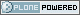LACSI Collaborators include: